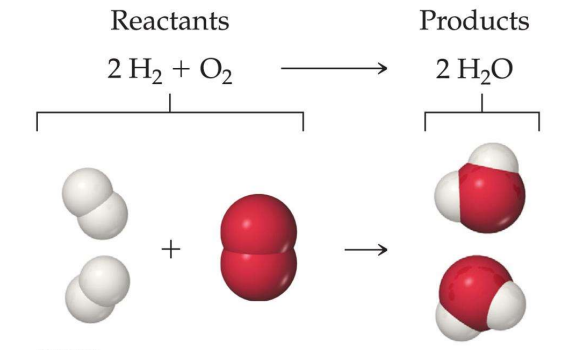HS-PS1-5

# Limiting Reagent Study Guide

A reagent that is totally consumed in a reaction is a limiting reagent. The amount of product is decided by limiting the reagent.

Table of content

## INTRODUCTION

Imagine you were making pasta. You have chopped the vegetables, made the sauce, and were about to boil some pasta when you realize that the pasta is present in half the amount than you required. What will you do?

You will make the pasta that is available only. This is exactly what happens during a chemical reaction when one of the reagents is in a limited amount. Let’s understand the limiting reagent and which reactant is the limiting reactant a bit more!

### LIMITING REAGENT

The limiting reagent is a reactant that is totally consumed in a reaction. In simple words, the reagent limits the reaction as the amount of that reactant is limited.2H₂ + O₂ → 2H₂O

Suppose we take 100 grams of H₂ and O₂ each. Our very first instinct would be that both of the reactants are in equal amounts. But are they in equal amounts?

No! 100 grams of O₂ means 3.125 moles of O₂ are there, while H₂ present is 50 moles.

### HOW IS THIS HAPPENING?

By use of a simple formula:

Number of moles = Given mass/ Molecular mass

The molecular mass of O₂ is 32 g/mol, while that of H₂ is 2 g/mol. Therefore, the number of moles of O₂ is 100/32 = 3.125 moles. And for H₂, the number of moles would be 50.

For the above reaction, the number of moles of H₂ required is twice O₂. This implies 3.125 x 2 moles of H₂ would be required, that is 6.25 moles.

The remaining moles of H₂ cannot react any further because the other reactant, O₂, is present in a limited amount. Because of that, O₂ limited is called a limiting reagent, and due to its limited amount, the reaction stops after it is fully consumed.

### How to Find a Limiting Reagent?

L.R. = n/S.C.

L.R. denotes the limiting reagent, n is the mole, and S.C. is the stoichiometric ratio. Whichever reagent's mole to stoichiometric ratio is the lowest will be our limiting reagent.

Let us get a better understanding with the help of an example.

Question. 1 g of H2 gas reacts with 1 g of Cl2 gas. Determine the product's limiting reagent.

Answer. Firstly, write down the reaction.

H2 + Cl2 → 2HCl

There are two reactants in the above reaction, and we have to identify the limiting reagent among these two reactants because the limiting reagent determines the final product.

Let us now find out mole to stoichiometric ratio of hydrogen. (n/SC)H2 =

First, we will find out the moles of hydrogen.

nH2 = W/M = 1 g/2 g per mole = ½ mole

W is the given mass, and M is the molar mass.

Next, we have to find out the stoichiometric ratio of hydrogen. S.C H2 = 1

Now we have to substitute the following in the formula to get the stoichiometric ratio of hydrogen. (n/S.C)H2 = ½ / 1 = ½

So the S.C. of hydrogen is ½

The same steps are required to determine the stoichiometric ratio of chlorine gas.

(n/S.C) Cl2 =

nCl2 = W/M = 1/71 moles

S.C Cl2 = 1

(n/S.C) Cl2 = 1/71

After finding out the stoichiometric ratio of both reactants, we find out that the mole to stoichiometric ratio of chlorine is the lowest. So the products limiting reagent is chlorine.

### WHAT IS THE USE OF THIS CONCEPT?

Now the whole reaction depends on the limiting reagent, and the amount of the product formed will be decided by the limiting reagent. For example, in this case, 2 moles of H₂O are produced when 1 mole of O₂ reacts with 2 moles of H₂. So, H₂O produced will also be dependent on the amount/ moles of O₂ present as O₂ is a limiting reagent. That means H₂O produced will be 3.125 x 2 moles = 6.25 moles.

## CONCLUSION

• The limiting reagent in a reaction is a reactant that is consumed in a reaction.
• Limiting reagents helps in finding the amount of product that will be formed in a reaction.

### FAQs

1. What is a limiting reagent?

The limiting reagent in a reaction is a reactant that is totally consumed in a reaction.

2. For the reaction N₂ 3H₂ → 2NH₃, if 1 mole of N₂ and 6 moles of H₂ are taken, which among the two will be limiting reagent?

N₂ + 3H₂ → 2NH₃

For 1 mole of N₂, 3 moles of H₂ are required. If we take 1 mole of N₂ and 6 moles of H₂, then N₂ will become a limiting reagent.

3. CH₄ + 2O₂ → CO₂ + 2H₂O is given. If we take 16 grams of CH₄ and 16 grams of O₂, then which one is a limiting reagent?

For 1 mole of CH₄, 2 moles of O₂ is required. If we take 16 grams of CH₄ and 16 grams of O₂, then O₂ will become a limiting reagent.

We hope you enjoyed studying this lesson and learned something cool about Limiting Reagent! Join our Discord community to get any questions you may have answered and to engage with other students just like you! Don't forget to download our app to experience our fun VR classrooms - we promise it makes studying much more fun! 😎